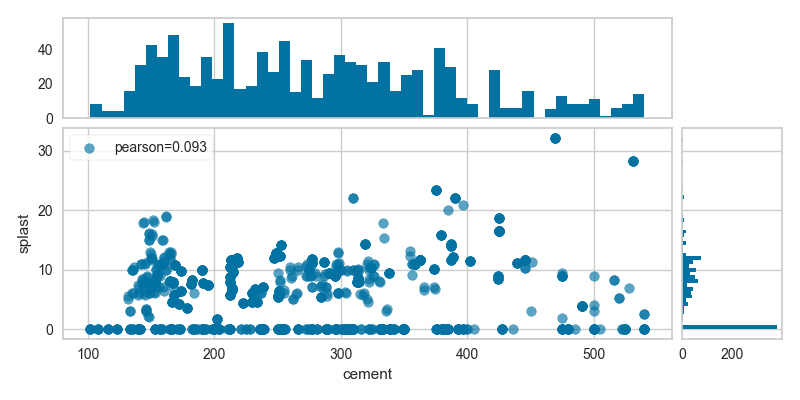# Direct Data Visualization¶

Sometimes for feature analysis you simply need a scatter plot to determine the distribution of data. Machine learning operates on high dimensional data, so the number of dimensions has to be filtered. As a result these visualizations are typically used as the base for larger visualizers; however you can also use them to quickly plot data during ML analysis.

## Joint Plot Visualization¶

A joint plot visualizer plots a feature against the target and shows the distribution of each via a histogram on each axis.

# Load the data
feature = "cement"
target = "strength"

# Get the X and y data from the DataFrame
X = df[feature]
y = df[target]

from yellowbrick.features import JointPlotVisualizer

visualizer = JointPlotVisualizer(feature=feature, target=target)

visualizer.fit(X, y)
visualizer.poof()The joint plot visualizer can also be plotted with hexbins in the case of many, many points.

visualizer = JointPlotVisualizer(
feature=feature, target=target, joint_plot='hex'
)

visualizer.fit(X, y)
visualizer.poof()## API Reference¶

class yellowbrick.features.jointplot.JointPlot(ax=None, columns=None, correlation='pearson', kind='scatter', hist=True, alpha=0.65, joint_kws=None, hist_kws=None, **kwargs)[source]

Bases: yellowbrick.features.base.FeatureVisualizer

Joint plots are useful for machine learning on multi-dimensional data, allowing for the visualization of complex interactions between different data dimensions, their varying distributions, and even their relationships to the target variable for prediction.

The Yellowbrick JointPlot can be used both for pairwise feature analysis and feature-to-target plots. For pairwise feature analysis, the columns argument can be used to specify the index of the two desired columns in X. If y is also specified, the plot can be colored with a heatmap or by class. For feature-to-target plots, the user can provide either X and y as 1D vectors, or a columns argument with an index to a single feature in X to be plotted against y.

Histograms can be included by setting the hist argument to True for a frequency distribution, or to "density" for a probability density function. Note that histograms requires matplotlib 2.0.2 or greater.

Parameters: ax : matplotlib Axes, default: None The axes to plot the figure on. If None is passed in the current axes will be used (or generated if required). This is considered the base axes where the the primary joint plot is drawn. It will be shifted and two additional axes added above (xhax) and to the right (yhax) if hist=True. columns : int, str, [int, int], [str, str], default: None Determines what data is plotted in the joint plot and acts as a selection index into the data passed to fit(X, y). This data therefore must be indexable by the column type (e.g. an int for a numpy array or a string for a DataFrame). If None is specified then either both X and y must be 1D vectors and they will be plotted against each other or X must be a 2D array with only 2 columns. If a single index is specified then the data is indexed as X[columns] and plotted jointly with the target variable, y. If two indices are specified then they are both selected from X, additionally in this case, if y is specified, then it is used to plot the color of points. Note that these names are also used as the x and y axes labels if they aren’t specified in the joint_kws argument. correlation : str, default: ‘pearson’ The algorithm used to compute the relationship between the variables in the joint plot, one of: ‘pearson’, ‘covariance’, ‘spearman’, ‘kendalltau’. kind : str in {‘scatter’, ‘hex’}, default: ‘scatter’ The type of plot to render in the joint axes. Note that when kind=’hex’ the target cannot be plotted by color. hist : {True, False, None, ‘density’, ‘frequency’}, default: True Draw histograms showing the distribution of the variables plotted jointly. If set to ‘density’, the probability density function will be plotted. If set to True or ‘frequency’ then the frequency will be plotted. Requires Matplotlib >= 2.0.2. alpha : float, default: 0.65 Specify a transparency where 1 is completely opaque and 0 is completely transparent. This property makes densely clustered points more visible. {joint, hist}_kws : dict, default: None Additional keyword arguments for the plot components. kwargs : dict Keyword arguments that are passed to the base class and may influence the visualization as defined in other Visualizers.

Examples

>>> viz = JointPlot(columns=["temp", "humidity"])
>>> viz.fit(X, y)
>>> viz.poof()

Attributes: corr_ : float The correlation or relationship of the data in the joint plot, specified by the correlation algorithm.
correlation_methods = {'covariance': <function JointPlot.<lambda>>, 'kendalltau': <function JointPlot.<lambda>>, 'pearson': <function JointPlot.<lambda>>, 'spearman': <function JointPlot.<lambda>>}
draw(self, x, y, xlabel=None, ylabel=None)[source]

Draw the joint plot for the data in x and y.

Parameters: x, y : 1D array-like The data to plot for the x axis and the y axis xlabel, ylabel : str The labels for the x and y axes.
finalize(self, **kwargs)[source]

Finalize executes any remaining image modifications making it ready to show.

fit(self, X, y=None)[source]

Fits the JointPlot, creating a correlative visualization between the columns specified during initialization and the data and target passed into fit:

• If self.columns is None then X and y must both be specified as 1D arrays or X must be a 2D array with only 2 columns.
• If self.columns is a single int or str, that column is selected to be visualized against the target y.
• If self.columns is two ints or strs, those columns are visualized against each other. If y is specified then it is used to color the points.

This is the main entry point into the joint plot visualization.

Parameters: X : array-like An array-like object of either 1 or 2 dimensions depending on self.columns. Usually this is a 2D table with shape (n, m) y : array-like, default: None An vector or 1D array that has the same length as X. May be used to either directly plot data or to color data points.
xhax

The axes of the histogram for the top of the JointPlot (X-axis)

yhax

The axes of the histogram for the right of the JointPlot (Y-axis)